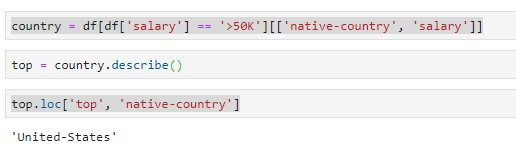# Demographic Data Analyzer Cert - Question about a task

Tell us what’s happening:
Question # 8: What country has the highest percentage of people that earn >50K and what is that percentage?

Into test_module.py “Iran” is the expected result but I´m getting “United-States” as a result running this code:Can someone clarify and help me?
Thanks!

Your code so far

country = df[df[‘salary’] == ‘>50K’][[‘native-country’, ‘salary’]]
top = country.describe()
top.loc[‘top’, ‘native-country’]

User Agent is: `Mozilla/5.0 (Windows NT 10.0; Win64; x64) AppleWebKit/537.36 (KHTML, like Gecko) Chrome/84.0.4147.89 Safari/537.36`.

Challenge: Demographic Data Analyzer

Link to the challenge:

Nevermind, I was misunderstanding the task…

@cordonidamian can you share the solution here

please share the solution if you can…

Hi! I got the solution running this code:

3 Likes

Thanks @cordonidamian you are a life saver. Hope we can help each other in future regarding Data Analysis projects.

I tried this method, for some reason, I am getting ‘?’ for country and NaN for value. Did you clean up the data beforehand?

This is my solution using groupby:

``````# Defining the new columns in a new grouped table
aggregation = {
'>50K':  ('salary', lambda x: (x == ">50K").sum()),
'<=50K': ('salary', lambda x: (x == "<=50K").sum()),
}

# Creating a new table that has native-country as the index and columns that have the counts of >50K and <=50K
df2 = df.groupby('native-country').agg(**aggregation)

# Function that gets the total per country
def getTotal(row):
return row['>50K'] + row['<=50K']

# Add a column that calculate the % of those that earn >50K
df2['>50K%'] = df2.apply(lambda row: (row['>50K'] / getTotal(row) *100).round(1), axis=1)

# Sorting the values by >50K% - largest number at the top
df2 = df2.sort_values(">50K%",ascending=False)

# Get the name of the country and put into Title Case
highest_earning_country = df2.iloc.name.title()

# Get the percentage
highest_earning_country_percentage = df2.iloc[">50K%"]
``````
1 Like